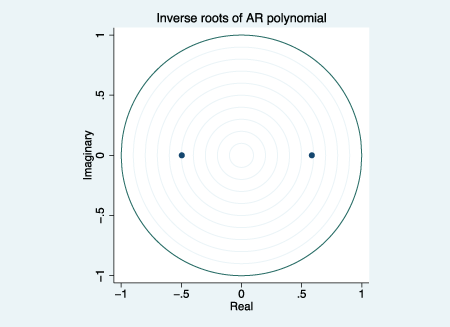»  Home »  Products »  Training »  NetCourses »  NetCourseNow »  NCNow461: Univariate time series with StataTraining

## NetCourseNow™ 461: Univariate time series with Stata

\$495

Discounts available for enrollments of
two or more participants.

NetCourseNow is a self-paced, personalized learning experience. All lessons will be posted at once, and you will be given the email address of your personal NetCourse instructor, to whom you can email questions about the lessons. Enroll now, and begin when you're ready.

Content:
Learn about univariate time-series analysis with an emphasis on the practical aspects most needed by practitioners and applied researchers. Written for a broad array of users, including economists, forecasters, financial analysts, managers, and anyone who wants to analyze time-series data. Become expert in handling date and date-time data; time-series operators; time-series graphics, basic forecasting methods; ARIMA, ARMAX, and seasonal models.

Prerequisites:

• Stata 17 or Stata 16, installed and working
• Course content of NetCourse 101 or equivalent knowledge
• Familiarity with basic cross-sectional summary statistics and linear regression
• Internet web browser, installed and working
(course is platform independent)

#### Course content

Lesson 1: Introduction

• Time-series data in Stata
• Working with dates
• Time-series operators
• Drawing graphs
• Simple smoothers and forecasting techniques
• Moving averages
• Exponential smoothers
• Holt–Winters forecasting

Lesson 2: Descriptive analysis of time series

• The nature of time series
• Autocorrelation
• White noise
• Stationarity
• Time-series processes
• Moving average (MA)
• Autoregressive (AR)
• Mixed autoregressive moving average (ARMA)
• The sample autocorrelation and partial autocorrelation functions
• Introduction to spectral analysis—the periodogram

Lesson 3: Forecasting II: ARIMA and ARMAX models

• Basic ARIMA models
• Using ARMA processes to model series
• Choosing the number of AR and MA terms
• Selecting the best model from information criteria
• Forecasting
• Seasonal ARIMA models
• Models with exogenous regressors—ARMAX models
• A brief tour of intervention analysis
• Level shifts

Lesson 4: Regression analysis of time-series data

• Basic regression analysis
• Autocorrelation
• The Durbin–Watson test
• Other tests for autocorrelation
• Estimation with autocorrelated errors
• The Newey–West covariance matrix estimator
• ARMAX estimation
• Cochrane–Orcutt and Prais–Winsten methods
• Lagged dependent variables as regressors
• Dummy variables and additive seasonal effects
• Test for structural break
• Nonstationary series and OLS regression
• Unit-root processes
• ARCH
• A simple ARCH model
• Testing for ARCH
• GARCH models
• Extensions
• Markov-switching models
• Markov-switching dynamic regression
• Markov-switching autoregression
• Threshold regression
• A self-exciting threshold model
• A second threshold model
• Letting threshold choose the number of regimes

The previous four lessons constitute the core material of the course. The following lesson is optional and introduces Stata's multivariate time-series capabilities.

Bonus lesson: Overview of multivariate time-series analysis using Stata

• Vector autoregressive (VAR) models
• Estimating VAR models
• Impulse–response analysis
• Forecasting
• Structural VARs
• Cointegration
• Testing for cointegration
• Vector error-correction (VEC) models